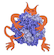IMP  2.4.0 The Integrative Modeling Platform
algebra.h
Go to the documentation of this file.
1 /**
2  * \file IMP/algebra.h
3  * \brief Include all non-deprecated headers in IMP.algebra.
4  *
6  */
7
8 #ifndef IMP_ALGEBRA_H
9 #define IMP_ALGEBRA_H
11 #include <IMP/algebra/Cone3D.h>
12 #include <IMP/algebra/Cylinder3D.h>
14 #include <IMP/algebra/Gaussian3D.h>
16 #include <IMP/algebra/GridD.h>
17 #include <IMP/algebra/LinearFit.h>
19 #include <IMP/algebra/Plane3D.h>
22 #include <IMP/algebra/Rotation2D.h>
23 #include <IMP/algebra/Rotation3D.h>
24 #include <IMP/algebra/Segment3D.h>
25 #include <IMP/algebra/Sphere3D.h>
26 #include <IMP/algebra/SphereD.h>
31 #include <IMP/algebra/Triangle3D.h>
32 #include <IMP/algebra/Vector2D.h>
33 #include <IMP/algebra/Vector3D.h>
35 #include <IMP/algebra/VectorD.h>
38 #include <IMP/algebra/constants.h>
39 #include <IMP/algebra/distance.h>
41 #include <IMP/algebra/endian.h>
49 #include <IMP/algebra/io.h>
53 #include <IMP/algebra/utility.h>
57 #endif /* IMP_ALGEBRA_H */
Basic types used by IMP.
Simple 3D sphere patch class.
A class to represent a voxel grid.
Classes and operations related with rotations.
A class to represent a voxel grid.
Linear fit of data points.
stores a cylinder
A class to represent a voxel grid.
Simple 2D vector class.
Simple 3D sphere class.
Simple 3D ellipsoid class.
Simple implementation of segments in 3D.
functions to deal with endian of EM images
Functions to deal with very common math operations.
Functions to generate vectors.
A class to represent a voxel grid.
A bounding box in D dimensions.
Generate surface for a set of atoms.
Functions to generate vectors.
Simple D vector class.
Stores and converts spherical coordinates.
Represent a triangle in 3D.
Various useful constants.
Simple 3D rotation class.
Simple 3D transformation class.
Simple D vector class.
Simple 3D plane class.
Functionality only available in Python.
align sets of points.
Classes to write entities in algebra to files.
Represent a cone in 3D.
fit the data with parabola
Simple 3D vector class.
A class to represent a voxel grid.
All grids that are in the Python API should be defined here.
Reflect about a plane in 3D.
Functions to generate vectors.
Simple 3D rotation class.
Gaussian shape.
Principal component analysis of a set of points.
Simple 3D sphere class.
Various important macros for implementing geometry.
Various important macros for implementing geometry.
distance metrics
A class to represent a voxel grid.